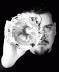## Testing Random Dice Rolls

I've decided to rewrite my Perl testing course from scratch and hit upon an interesting problem that is outside of the scope of the course, but is a perfect fit for this site: testing dice rolls. I have the the following function as part of a student lesson in the course:

``````sub roll_dice {
my \$arg_for = shift;

my \$sides = \$arg_for->{sides} || 6;
my \$times = \$arg_for->{times} || 1;

# we could have also chosen to croak() or throw an exception here
\$sides = 6 if \$sides < 2;
\$times = 1 if \$times < 1;

return sum( map { 1 + int rand \$sides } 1 .. \$times );  # fixed!
}
``````

Rolling dice is random and it's fair to say that testing randomness is hard. Is the above correct? For the purposes of the tests, students only need to test if the expected values are in range. But what about the distribution of those values?

Update: What an irony that I had a small bug that would pass "range" tests, but not a chi-square test :)

Let's say I roll a six-sided die 60 times and I get the following results for the numbers 1 through 6, respectively:

``````16, 5, 9, 7, 6, 17
``````

Is that really random? It doesn't look like it. In fact, if you have dice at home, there's a good chance that they will also give you a non-random distribution. Dice often have the pips formed by a dimple in the surface. This means that the face with 1 pip weighs more than the face with 6 pips. This will skew your results in the long-term and that's why dice often have the pips printed rather than gouged out.

For the above distribution, though, you could use Pearson's chi-square test. In Perl, it's available via Statistics::ChiSquare:

``````use Statistics::ChiSquare 'chisquare';
say chisquare( 16, 5, 9, 7, 6, 17 );
``````

Unfortunately, that prints:

`    There's a >1% chance, and a <5% chance, that this data is random.`

As it turns out, the chi-square test says there's only a 1.8% chance of those numbers being "fair". So not only is the interface awkward, the numbers are rounded off to the point where they're not very useful.

What's worse, it doesn't handle non-uniform distributions. Let's say I wanted to compute the odds of something being amiss when I rolled two-six sided dice. The frequency looks like this:

``````Sum   Frequency   Relative Frequency
2     1           1/36
3     2           2/36
4     3           3/36
5     4           4/36
6     5           5/36
7     6           6/36
8     5           5/36
9     4           4/36
10    3           3/36
11    2           2/36
12    1           1/36
``````

Statistics::ChiSquare used to have a chisquare_nonuniform() function, but that was removed years ago (I don't know why).

So that's two strikes against using this module.

What I want is a way of taking a list of actual die rolls and a list of expected die rolls and calculating the probability that the distribution is fair. I initially wrote the code for this, but made a small math error in calculating chi-square. amon on stackoverflow presented the correct algorithm.

``````use Carp;
use List::Util qw< sum >;
use Statistics::Distributions qw< chisqrprob >;

sub chi_squared_test {
my %arg_for = @_;
my \$observed = \$arg_for{observed}
// croak q(Argument "observed" required);
my \$expected = \$arg_for{expected}
// croak q(Argument "expected" required);

@\$observed == @\$expected or croak q(Input arrays must have same length);

my \$chi_squared = sum map {
( \$observed->[\$_] - \$expected->[\$_] )**2
/
\$expected->[\$_]
} 0 .. \$#\$observed;

my \$degrees_of_freedom = @\$observed - 1;
my \$probability        = chisqrprob(
\$degrees_of_freedom,
\$chi_squared
);

return \$probability;
}
``````

With that, now I can just do this:

``````say chi_squared_test(
observed => [ 16, 5, 9, 7, 6, 17 ],
expected => [ (10) x 6 ]
);
``````

And that prints out `0.018360`, the probability of that set of die rolls occurring by chance.

Building up our `expected` list is left as an exercise for the reader.

Insubstantial nitpick: Shouldn't

`return sum( ( 1 + int( rand(\$sides) ) ) x \$times );`

be

`return sum( map { 1 + int rand \$sides } 1 .. \$times );`

? The `x` operator repeats a value, not an expression. Therefore the original code is equivalent to

`return \$times * int rand \$sides;`Freelance Perl/Testing/Agile consultant and trainer. See http://www.allaroundtheworld.fr/ for our services. If you have a problem with Perl, we will solve it for you. And don't forget to buy my book! http://www.amazon.com/Beginning-Perl-Curtis-Poe/dp/1118013840/